## Current and resistance

7-12-99

### Electrical resistance

Voltage can be thought of as the pressure pushing charges along a conductor, while the electrical resistance of a conductor is a measure of how difficult it is to push the charges along. Using the flow analogy, electrical resistance is similar to friction. For water flowing through a pipe, a long narrow pipe provides more resistance to the flow than does a short fat pipe. The same applies for flowing currents: long thin wires provide more resistance than do short thick wires.

The resistance (R) of a material depends on its length, cross-sectional area, and the resistivity (the Greek letter rho), a number that depends on the material: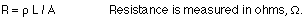The resistivity and conductivity are inversely related. Good conductors have low resistivity, while poor conductors (insulators) have resistivities that can be 20 orders of magnitude larger.

Resistance also depends on temperature, usually increasing as the temperature increases. For reasonably small changes in temperature, the change in resistivity, and therefore the change in resistance, is proportional to the temperature change. This is reflected in the equations:At low temperatures some materials, known as superconductors, have no resistance at all. Resistance in wires produces a loss of energy (usually in the form of heat), so materials with no resistance produce no energy loss when currents pass through them.

### Ohm's Law

In many materials, the voltage and resistance are connected by Ohm's Law:

Ohm's Law : V = IR

The connection between voltage and resistance can be more complicated in some materials.These materials are called non-ohmic. We'll focus mainly on ohmic materials for now, those obeying Ohm's Law.

### Example

A copper wire has a length of 160 m and a diameter of 1.00 mm. If the wire is connected to a 1.5-volt battery, how much current flows through the wire?

The current can be found from Ohm's Law, V = IR. The V is the battery voltage, so if R can be determined then the current can be calculated. The first step, then, is to find the resistance of the wire:L is the length, 1.60 m. The resistivity can be found from the table on page 535 in the textbook.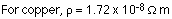The area is the cross-sectional area of the wire. This can be calculated using: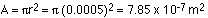The resistance of the wire is then: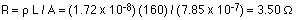The current can now be found from Ohm's Law:

I = V / R = 1.5 / 3.5 = 0.428 A

### Electric power

Power is the rate at which work is done. It has units of Watts. 1 W = 1 J/s

Electric power is given by the equations: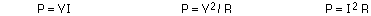The power supplied to a circuit by a battery is calculated using P = VI.

Batteries and power supplies supply power to a circuit, and this power is used up by motors as well as by anything that has resistance. The power dissipated in a resistor goes into heating the resistor; this is know as Joule heating. In many cases, Joule heating is wasted energy. In some cases, however, Joule heating is exploited as a source of heat, such as in a toaster or an electric heater.

The electric company bills not for power but for energy, using units of kilowatt-hours.

1 kW-h = 3.6 x 106 J

One kW-h typically costs about 10 cents, which is really quite cheap. It does add up, though. The following equation gives the total cost of operating something electrical:

Cost = (Power rating in kW) x (number of hours it's running) x (cost per kW-h)

An example...if a 100 W light bulb is on for two hours each day, and energy costs \$0.10 per kW-h, how much does it cost to run the bulb for a month?

Cost = 0.1 kW x 60 hours x \$0.1/kW-h = \$0.6, or 60 cents.

Try this at home - figure out the monthly cost of using a particular appliance you use every day. Possibilities include hair dryers, microwaves, TV's, etc. The power rating of an appliance like a TV is usually written on the back, and if it doesn't give the power it should give the current. Anything you plug into a wall socket runs at 120 V, so if you know that and the current you can figure out how much power it uses.

The cost for power that comes from a wall socket is relatively cheap. On the other hand, the cost of battery power is much higher. \$100 per kW-h, a thousand times more than what it costs for AC power from the wall socket, is a typical value.

Although power is cheap, it is not limitless. Electricity use continues to increase, so it is important to use energy more efficiently to offset consumption. Appliances that use energy most efficiently sometimes cost more but in the long run, when the energy savings are accounted for, they can end up being the cheaper alternative.

### Direct current (DC) vs. alternating current (AC)

A battery produces direct current; the battery voltage (or emf) is constant, which generally results in a constant current flowing one way around a circuit. If the circuit has capacitors, which store charge, the current may not be constant, but it will still flow in one direction. The current that comes from a wall socket, on the other hand, is alternating current. With alternating current, the current continually changes direction. This is because the voltage (emf) is following a sine wave oscillation. For a wall socket in North America, the voltage changes from positive to negative and back again 60 times each second.

If you look at the voltage at its peak, it hits about +170 V, decreases through 0 to -170 V, and then rises back through 0 to +170 V again. (You might think this value of 170 V should really be 110 - 120 volts. That's actually a kind of average of the voltage, but the peak really is about 170 V.) This oscillating voltage produces an oscillating electric field; the electrons respond to this oscillating field and oscillate back and forth, producing an oscillating current in the circuit.The graph above shows voltage as a function of time, but it could just as well show current as a function of time: the current also oscillates at the same frequency.

### Root mean square

This average value we use for the voltage from a wall socket is known as the root mean square, or rms, average. Because the voltage varies sinusoidally, with as much positive as negative, doing a straight average would get you zero for the average voltage. The rms value, however, is obtained in this way:

• first, square everything (this makes everything positive)
• second, average
• third, take the square root of the average

Here's an example, using the four numbers -1, 1, 3, and 5. The average of these numbers is 8 / 4 = 2. To find the rms average, you square everything to get 1, 1, 9, and 25. Now you average those values, obtaining 36 / 4 = 9. Finally, take the square root to get 3. The average is 2, but the rms average is 3.

Doing this for a sine wave gets you an rms average that is the peak value of the sine wave divided by the square root of two. This is the same as multiplying by 0.707, so the relationship between rms values and peak values for voltage and current is:

Vrms = 0.707 Vo and Irms = 0.707 Io

In North America, the rms voltage is about 120 volts. If you need to know about the average power used, it is the rms values that go into the calculation.

### Series circuits

A series circuit is a circuit in which resistors are arranged in a chain, so the current has only one path to take. The current is the same through each resistor. The total resistance of the circuit is found by simply adding up the resistance values of the individual resistors:

equivalent resistance of resistors in series : R = R1 + R2 + R3 + ...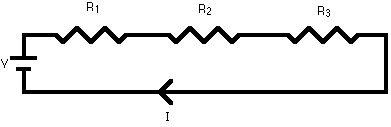A series circuit is shown in the diagram above. The current flows through each resistor in turn. If the values of the three resistors are: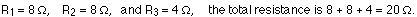With a 10 V battery, by V = I R the total current in the circuit is:

I = V / R = 10 / 20 = 0.5 A. The current through each resistor would be 0.5 A.

### Parallel circuits

A parallel circuit is a circuit in which the resistors are arranged with their heads connected together, and their tails connected together. The current in a parallel circuit breaks up, with some flowing along each parallel branch and re-combining when the branches meet again. The voltage across each resistor in parallel is the same.

The total resistance of a set of resistors in parallel is found by adding up the reciprocals of the resistance values, and then taking the reciprocal of the total:

equivalent resistance of resistors in parallel: 1 / R = 1 / R1 + 1 / R2 + 1 / R3 +...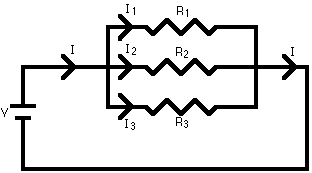A parallel circuit is shown in the diagram above. In this case the current supplied by the battery splits up, and the amount going through each resistor depends on the resistance. If the values of the three resistors are:With a 10 V battery, by V = I R the total current in the circuit is: I = V / R = 10 / 2 = 5 A.

The individual currents can also be found using I = V / R. The voltage across each resistor is 10 V, so:

I1 = 10 / 8 = 1.25 A
I2 = 10 / 8 = 1.25 A
I3=10 / 4 = 2.5 A

Note that the currents add together to 5A, the total current.

### A parallel resistor short-cut

If the resistors in parallel are identical, it can be very easy to work out the equivalent resistance. In this case the equivalent resistance of N identical resistors is the resistance of one resistor divided by N, the number of resistors. So, two 40-ohm resistors in parallel are equivalent to one 20-ohm resistor; five 50-ohm resistors in parallel are equivalent to one 10-ohm resistor, etc.

When calculating the equivalent resistance of a set of parallel resistors, people often forget to flip the 1/R upside down, putting 1/5 of an ohm instead of 5 ohms, for instance. Here's a way to check your answer. If you have two or more resistors in parallel, look for the one with the smallest resistance. The equivalent resistance will always be between the smallest resistance divided by the number of resistors, and the smallest resistance. Here's an example.

You have three resistors in parallel, with values 6 ohms, 9 ohms, and 18 ohms. The smallest resistance is 6 ohms, so the equivalent resistance must be between 2 ohms and 6 ohms (2 = 6 /3, where 3 is the number of resistors).

Doing the calculation gives 1/6 + 1/12 + 1/18 = 6/18. Flipping this upside down gives 18/6 = 3 ohms, which is certainly between 2 and 6.

### Circuits with series and parallel components

Many circuits have a combination of series and parallel resistors. Generally, the total resistance in a circuit like this is found by reducing the different series and parallel combinations step-by-step to end up with a single equivalent resistance for the circuit. This allows the current to be determined easily. The current flowing through each resistor can then be found by undoing the reduction process.

General rules for doing the reduction process include:

1. Two (or more) resistors with their heads directly connected together and their tails directly connected together are in parallel, and they can be reduced to one resistor using the equivalent resistance equation for resistors in parallel.

2. Two resistors connected together so that the tail of one is connected to the head of the next, with no other path for the current to take along the line connecting them, are in series and can be reduced to one equivalent resistor.

Finally, remember that for resistors in series, the current is the same for each resistor, and for resistors in parallel, the voltage is the same for each one.